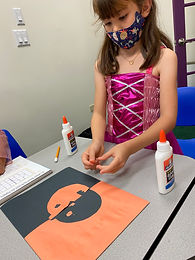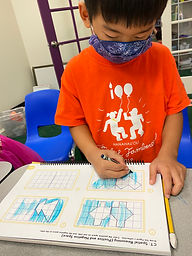## Ms. Brianne

### Target 1​

###### Lesson Type:

New

Number Operation

:

Computation

Solve multi-step word problems within 100 (addition, subtraction, multiplication, and division).

###### 1:

Identify the correct arithmetic processes based on the information presented in word problems.

###### 2:

Find the key words in word problems that indicate the correct arithmetic process to use.

###### 3:

Find and use the needed information in a word problem in order to solve.

3rd

###### Vocabulary:

Activities:

• Students read and made sense of word problems. They chose the appropriate operation and used it to solve the problem.
• Students reviewed different strategies of how to solve multiplication and division problems.### Home Exploration

###### Guiding Questions:## Absent Students:

Max

### Target 2

:

###### 1:

Understand how perception of a figure is comprised of an interaction between positive space (the figure) and negative space (the background).

###### Vocabulary:

Negative, Positive, Symmetrical, Line of Symmetry

Activities:

• Students were introduced to positive and negative space.
• Students drew a reflection for a given image across a line of symmetry. Then they colored one half using positive space and the other half using negative space.
• Students created their own jack o lanterns using positive and negative space.### Home Exploration

###### Guiding Questions:### Target 3

:

###### Vocabulary:

Activities:### Home Exploration